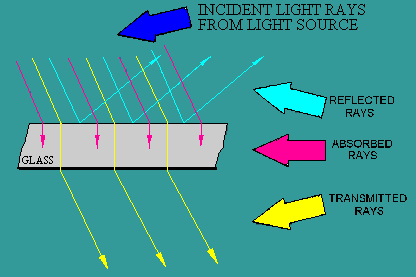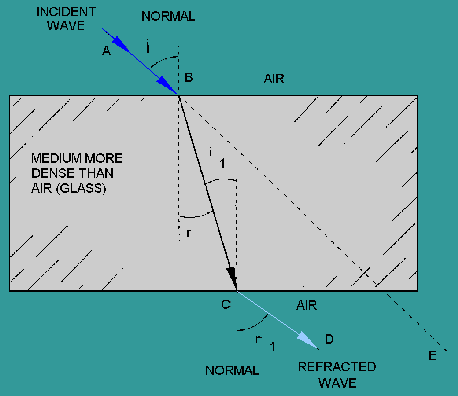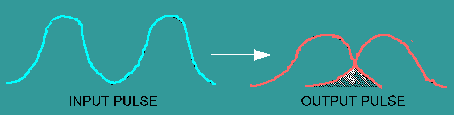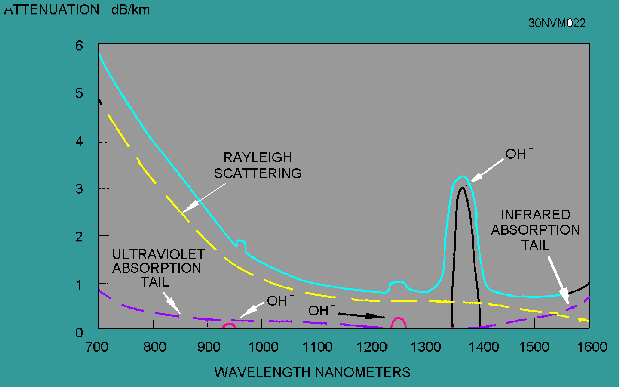Custom SearchSUMMARY Now that you have completed this chapter, let's review some of the new terms, concepts, and ideas that you have learned. You should have a thorough understanding of these principles before moving on to chapter 3. A LIGHT WAVE is a form of energy that is moved by wave motion. WAVE MOTION is defined as a recurring disturbance advancing through space with or without the use of a physical medium. SCIENTIFIC EXPERIMENTS seem to show that light is composed of tiny particles, while other experiments indicate that light is made up of waves. Today, physicists have come to accept a theory concerning light that is a combination of particle (ray) theory and wave (mode) theory. TRANSVERSE WAVE MOTION describes the up and down wave motion that is at right angle (transverse) to the outward motion of the waves. LIGHT RAYS, when they encounter any substance, are either transmitted, refracted, reflected, or absorbed.REFLECTION occurs when a wave strikes an object and bounces back (toward the source). The wave that moves from the source to the object is called the incident wave , and the wave that moves away from the object is called the reflected wave.The LAW OF REFLECTION states that the angle of incidence is equal to the angle of reflection. REFRACTION occurs when a wave traveling through two different mediums passes through the boundary of the mediums and bends toward or away from the normal.The RAY THEORY and the MODE THEORY describe how light energy is transmitted along an optical fiber. The INDEX OF REFRACTION is the basic optical material property that measures the speed of light in an optical medium. SNELL'S LAW OF REFRACTION describes the relationship between the incident and the refracted rays when light rays encounter the boundary between two different transparent materials. TOTAL INTERNAL REFLECTION occurs when light rays are totally reflected at the boundary between two different transparent materials. The angle at which total internal reflection occurs is called the critical angle of incidence. The CORE, CLADDING, and COATING or BUFFER are the three basic parts of an optical fiber.The RAY THEORY describes how light rays propagate along an optical fiber. MERIDIONAL RAYS pass through the axis of the optical fiber. SKEW RAYS propagate through an optical fiber without passing through its axis. BOUND RAYS propagate through an optical fiber core by total internal reflection. UNBOUND RAYS refract out of the fiber core into the cladding and are eventually lost.The ACCEPTANCE ANGLE is the maximum angle to the axis of the fiber that light entering the fiber is bound or propagated. The light ray incident on the fiber core must be within the acceptance cone defined by the acceptance angle to be propagated along an optical fiber.NUMERICAL APERTURE (NA) is a measurement of the ability of an optical fiber to capture light. The MODE THEORY uses electromagnetic wave behavior to describe the propagation of light along an optical fiber. A set of guided electromagnetic waves are called the modes of the fiber. MODES traveling in an optical fiber are said to be transverse. Modes are described by their electric, E(x,y,z), and magnetic, H(x,y,z), fields. The electric field and magnetic field are at right angles to each other. NORMALIZED FREQUENCY determines how many modes a fiber can support. The number of modes is represented by the normalized frequency constant. SINGLE MODE and MULTIMODE FIBERS are classified by the number of modes that propagate along the optical fiber. Single mode fibers propagate only one mode because the core size approaches the operational wavelength. Multimode fibers can propagate over 100 modes depending on the core size and numerical aperture. ATTENUATION is the loss of optical power as light travels along an optical fiber. Attenuation in an optical fiber is caused by absorption, scattering, and bending losses. DISPERSION spreads the optical pulse as it travels along the fiber. Dispersion limits how fast information is transferred.ABSORPTION is the conversion of optical power into another energy form, such as heat. INTRINSIC ABSORPTION is caused by basic fiber-material properties. EXTRINSIC ABSORPTION is caused by impurities introduced into the fiber material. SILICA FIBERS are predominately used in fiber optic communications. They have low intrinsic material absorption at the wavelengths of operation. The WAVELENGTH OF OPERATION in fiber optics is between 700 nm and 1600 nm. The wavelength of operation is between the ultraviolet (below 400 nm) and infrared (above 2000 nm) intrinsic absorption regions. EXTRINSIC ABSORPTION occurs when impurities, such as hydroxyl ions (OH-), are introduced into the fiber. OH- absorption peaks define three regions or windows of preferred operation. The first window is centered at 850 nm. The second window is centered at 1300 nm. The third window is centered at 1550 nm.SCATTERING losses are caused by the interaction of light with density fluctuations within a fiber. Rayleigh scattering is the main source of loss in commercial fibers operating between 700 nm and 1600 nm. MICROBENDS are small microscopic bends of the fiber axis that occur mainly when a fiber is cabled. MACROBENDS are bends having a large radius of curvature relative to the fiber diameter. INTRAMODAL, or CHROMATIC, DISPERSION occurs because light travels through different materials and different waveguide structures at different speeds. MATERIAL DISPERSION is dependent on the light wavelengths interaction with the refractive index of the core. WAVEGUIDE DISPERSION is a function of the size of the fiber's core relative to the wavelength of operation. INTERMODAL, or MODAL, DISPERSION occurs because each mode travels a different distance over the same time span.Integrated Publishing, Inc. - A (SDVOSB) Service Disabled Veteran Owned Small Business